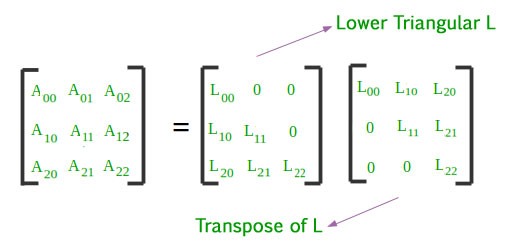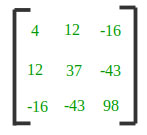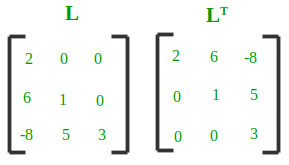Skip to content
Related Articles
Cholesky Decomposition : Matrix Decomposition
• Last Updated : 21 May, 2021

In linear algebra, a matrix decomposition or matrix factorization is a factorization of a matrix into a product of matrices. There are many different matrix decompositions. One of them is Cholesky Decomposition.
The Cholesky decomposition or Cholesky factorization is a decomposition of a Hermitian, positive-definite matrix into the product of a lower triangular matrix and its conjugate transpose. The Cholesky decomposition is roughly twice as efficient as the LU decomposition for solving systems of linear equations.
The Cholesky decomposition of a Hermitian positive-definite matrix A is a decomposition of the form A = [L][L]T, where L is a lower triangular matrix with real and positive diagonal entries, and LT denotes the conjugate transpose of L. Every Hermitian positive-definite matrix (and thus also every real-valued symmetric positive-definite matrix) has a unique Cholesky decomposition.Every symmetric, positive definite matrix A can be decomposed into a product of a unique lower triangular matrix L and its transpose: A = L LT
The following formulas are obtained by solving above lower triangular matrix and its transpose. These are the basis of Cholesky Decomposition Algorithm :[Tex]L_{i,j}=\frac{1}{L_{j,j}}(A_{i,j}-\sum_{k=0}^{j-1}L_{i,k}L_{j,k})     [/Tex]

Example

Input :Output :Below is the implementation of Cholesky Decomposition.

## C++

 // CPP program to decompose a matrix using// Cholesky Decomposition#include using namespace std; const int MAX = 100; void Cholesky_Decomposition(int matrix[][MAX],                                      int n){    int lower[n][n];    memset(lower, 0, sizeof(lower));     // Decomposing a matrix into Lower Triangular    for (int i = 0; i < n; i++) {        for (int j = 0; j <= i; j++) {            int sum = 0;             if (j == i) // summation for diagnols            {                for (int k = 0; k < j; k++)                    sum += pow(lower[j][k], 2);                lower[j][j] = sqrt(matrix[j][j] -                                        sum);            } else {                 // Evaluating L(i, j) using L(j, j)                for (int k = 0; k < j; k++)                    sum += (lower[i][k] * lower[j][k]);                lower[i][j] = (matrix[i][j] - sum) /                                      lower[j][j];            }        }    }     // Displaying Lower Triangular and its Transpose    cout << setw(6) << " Lower Triangular"          << setw(30) << "Transpose" << endl;    for (int i = 0; i < n; i++) {                 // Lower Triangular        for (int j = 0; j < n; j++)            cout << setw(6) << lower[i][j] << "\t";        cout << "\t";                 // Transpose of Lower Triangular        for (int j = 0; j < n; j++)            cout << setw(6) << lower[j][i] << "\t";        cout << endl;    }} // Driver Codeint main(){    int n = 3;    int matrix[][MAX] = { { 4, 12, -16 },                        { 12, 37, -43 },                        { -16, -43, 98 } };    Cholesky_Decomposition(matrix, n);    return 0;}

## Java

 // Java program to decompose// a matrix using Cholesky// Decomposition class GFG {     // static int MAX = 100;    static void Cholesky_Decomposition(int[][] matrix,                                       int n)    {        int[][] lower = new int[n][n];         // Decomposing a matrix        // into Lower Triangular        for (int i = 0; i < n; i++) {            for (int j = 0; j <= i; j++) {                int sum = 0;                 // summation for diagnols                if (j == i) {                    for (int k = 0; k < j; k++)                        sum += (int)Math.pow(lower[j][k],                                             2);                    lower[j][j] = (int)Math.sqrt(                        matrix[j][j] - sum);                }                 else {                     // Evaluating L(i, j)                    // using L(j, j)                    for (int k = 0; k < j; k++)                        sum += (lower[i][k] * lower[j][k]);                    lower[i][j] = (matrix[i][j] - sum)                                  / lower[j][j];                }            }        }         // Displaying Lower        // Triangular and its Transpose        System.out.println(" Lower Triangular\t Transpose");        for (int i = 0; i < n; i++) {             // Lower Triangular            for (int j = 0; j < n; j++)                System.out.print(lower[i][j] + "\t");            System.out.print("");             // Transpose of            // Lower Triangular            for (int j = 0; j < n; j++)                System.out.print(lower[j][i] + "\t");            System.out.println();        }    }     // Driver Code    public static void main(String[] args)    {        int n = 3;        int[][] matrix = new int[][] { { 4, 12, -16 },                                       { 12, 37, -43 },                                       { -16, -43, 98 } };         Cholesky_Decomposition(matrix, n);    }} // This code is contributed by mits

## Python3

 # Python3 program to decompose# a matrix using Cholesky# Decompositionimport mathMAX = 100; def Cholesky_Decomposition(matrix, n):     lower = [[0 for x in range(n + 1)]                for y in range(n + 1)];     # Decomposing a matrix    # into Lower Triangular    for i in range(n):        for j in range(i + 1):            sum1 = 0;             # sum1mation for diagnols            if (j == i):                for k in range(j):                    sum1 += pow(lower[j][k], 2);                lower[j][j] = int(math.sqrt(matrix[j][j] - sum1));            else:                                 # Evaluating L(i, j)                # using L(j, j)                for k in range(j):                    sum1 += (lower[i][k] *lower[j][k]);                if(lower[j][j] > 0):                    lower[i][j] = int((matrix[i][j] - sum1) /                                               lower[j][j]);     # Displaying Lower Triangular    # and its Transpose    print("Lower Triangular\t\tTranspose");    for i in range(n):                 # Lower Triangular        for j in range(n):            print(lower[i][j], end = "\t");        print("", end = "\t");                 # Transpose of        # Lower Triangular        for j in range(n):            print(lower[j][i], end = "\t");        print(""); # Driver Coden = 3;matrix = [[4, 12, -16],          [12, 37, -43],          [-16, -43, 98]];Cholesky_Decomposition(matrix, n); # This code is contributed by mits

## C#

 // C# program to decompose// a matrix using Cholesky// Decompositionusing System; class GFG {     // static int MAX = 100;    static void Cholesky_Decomposition(int[, ] matrix,                                       int n)    {        int[, ] lower = new int[n, n];         // Decomposing a matrix        // into Lower Triangular        for (int i = 0; i < n; i++) {            for (int j = 0; j <= i; j++) {                int sum = 0;                 // summation for diagnols                if (j == i) {                    for (int k = 0; k < j; k++)                        sum += (int)Math.Pow(lower[j, k],                                             2);                    lower[j, j] = (int)Math.Sqrt(                        matrix[j, j] - sum);                }                 else {                     // Evaluating L(i, j)                    // using L(j, j)                    for (int k = 0; k < j; k++)                        sum += (lower[i, k] * lower[j, k]);                    lower[i, j] = (matrix[i, j] - sum)                                  / lower[j, j];                }            }        }         // Displaying Lower        // Triangular and its Transpose        Console.WriteLine(            "  Lower Triangular\t   Transpose");        for (int i = 0; i < n; i++) {             // Lower Triangular            for (int j = 0; j < n; j++)                Console.Write(lower[i, j] + "\t");            Console.Write("");             // Transpose of            // Lower Triangular            for (int j = 0; j < n; j++)                Console.Write(lower[j, i] + "\t");            Console.WriteLine();        }    }     // Driver Code    static int Main()    {        int n = 3;        int[, ] matrix = { { 4, 12, -16 },                           { 12, 37, -43 },                           { -16, -43, 98 } };         Cholesky_Decomposition(matrix, n);        return 0;    }} // This code is contributed by mits

## PHP

 

## Javascript

 

Output:

Lower Triangular     Transpose
2  0  0              2  6  -8
6  1  0              0  1   5
-8 5  3              0  0   3    

References: Wikipedia – Cholesky decomposition
This article is contributed by Shubham Rana. If you like GeeksforGeeks and would like to contribute, you can also write an article using write.geeksforgeeks.org or mail your article to contribute@geeksforgeeks.org. See your article appearing on the GeeksforGeeks main page and help other Geeks.
Please write comments if you find anything incorrect, or you want to share more information about the topic discussed above.

Attention reader! Don’t stop learning now. Get hold of all the important mathematical concepts for competitive programming with the Essential Maths for CP Course at a student-friendly price. To complete your preparation from learning a language to DS Algo and many more,  please refer Complete Interview Preparation Course.

My Personal Notes arrow_drop_up